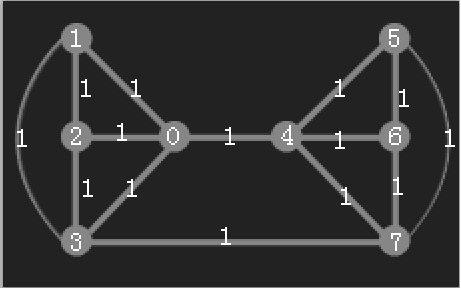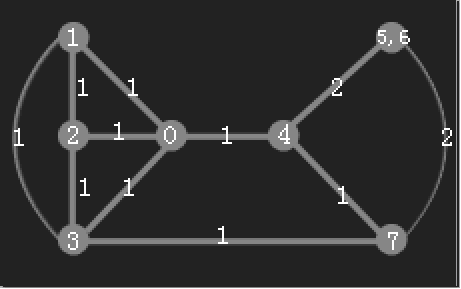# POJ 2914 Minimum Cut 题解 《挑战程序设计竞赛》POJ 2914 Minimum Cut

## 3.5借助水流解决问题的网络流

### 最小割

stoer_wagner就想着要偷懒啊，如果G的最小割Cut把G分成M，N两个点集，那么枚举的s和t无非以下两种情况：

①：如果s∈M，t∈N则Min-C（s，t）= Cut（这就是最终的最小割了）

②：如果s，t∈M（或者s，t∈N）则Min-C（s，t）<= Cut（那么合并s和t后并不影响最小割，所以就把st合并了得到中间结果图G'，继续在图G'上求最小割直到命中条件①1. 设最小割cut=INF, 任选一个点s到集合A中, 定义W(A, p)为A中的所有点到A外一点p的权总和.

2. 对刚才选定的s, 更新W(A,p)(该值递增).

3. 选出A外一点p, 且W(A,p)最大的作为新的s, 若A!=G(V), 则继续2.

4. 把最后进入A的两点记为s和t, 用W(A,t)更新cut.

5. 合并st，即新建顶点u, 边权w(u, v)=w(s, v)+w(t, v), 删除顶点s和t, 以及与它们相连的边.

6. 若|V|!=1则继续1.

```#include <iostream>
#include <cstdio>
#include <cstring>
#include <algorithm>
using namespace std;

#define MAX_N 500 + 16
#define INF 0x3f3f3f3f

int G[MAX_N][MAX_N];
int v[MAX_N];			//	v[i]代表节点i合并到的顶点
int w[MAX_N];			//	定义w(A,x) = ∑w(v[i],x)，v[i]∈A
bool visited[MAX_N];	//	用来标记是否该点加入了A集合

int stoer_wagner(int n)
{
int min_cut = INF;
for (int i = 0; i < n; ++i)
{
v[i] = i;		//	初始还未合并，都代表节点本身
}

while (n > 1)
{
int pre = 0;	//	pre用来表示之前加入A集合的点（在t之前一个加进去的点）
memset(visited, 0, sizeof(visited));
memset(w, 0, sizeof(w));
for (int i = 1; i < n; ++i)
{
int k = -1;
for (int j = 1; j < n; ++j)  //	选取V-A中的w(A,x)最大的点x加入集合
{
if (!visited[v[j]])
{
w[v[j]] += G[v[pre]][v[j]];
if (k == -1 || w[v[k]] < w[v[j]])
{
k = j;
}
}
}

visited[v[k]] = true;		//	标记该点x已经加入A集合
if (i == n - 1)				//	若|A|=|V|（所有点都加入了A），结束
{
const int s = v[pre], t = v[k];		//	令倒数第二个加入A的点（v[pre]）为s，最后一个加入A的点（v[k]）为t
min_cut = min(min_cut, w[t]);		//	则s-t最小割为w(A,t)，用其更新min_cut
for (int j = 0; j < n; ++j)			//	Contract(s, t)
{
G[s][v[j]] += G[v[j]][t];
G[v[j]][s] += G[v[j]][t];
}
v[k] = v[--n];						//	删除最后一个点（即删除t，也即将t合并到s）
}
// else 继续
pre = k;
}
}
return min_cut;
}

///////////////////////////SubMain//////////////////////////////////
int main(int argc, char *argv[])
{
#ifndef ONLINE_JUDGE
freopen("in.txt", "r", stdin);
freopen("out.txt", "w", stdout);
#endif
int n, m;
while (scanf("%d%d", &n, &m) != EOF)
{
memset(G, 0, sizeof(G));
while (m--)
{
int u, v, w;
scanf("%d%d%d", &u, &v, &w);
G[u][v] += w;
G[v][u] += w;
}
printf("%d\n", stoer_wagner(n));
}
#ifndef ONLINE_JUDGE
fclose(stdin);
fclose(stdout);
system("out.txt");
#endif
return 0;
}
///////////////////////////End Sub//////////////////////////////////```
 13771528 hankcs 2914 Accepted 1220K 3375MS C++ 1939B 2015-01-10 00:49:29

## Reference

http://blog.sina.com.cn/s/blog_700906660100v7vb.html

### 评论 2

1. #2

您好博主， 为什么是Min-C（s，t）<= Cut（s,t 属于 N）？我觉得应该是>=呀？

我就爱睡-7年前 (2017-01-27)回复
2. #1

用优先队列可以达到V*V*lgV

下雨了冒泡了9年前 (2015-05-08)回复